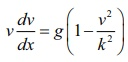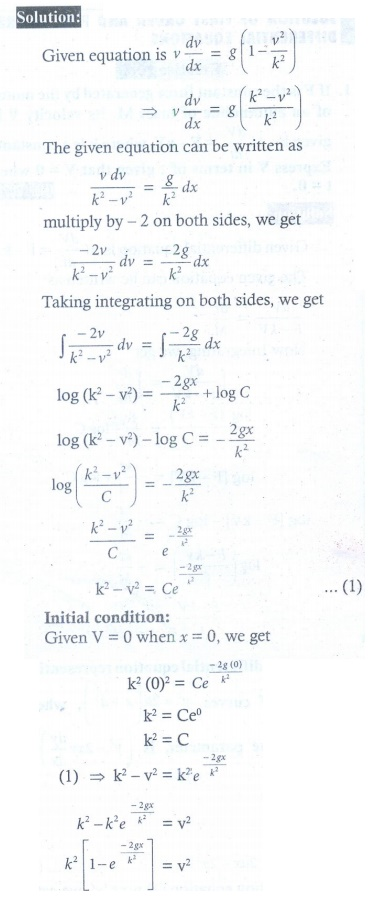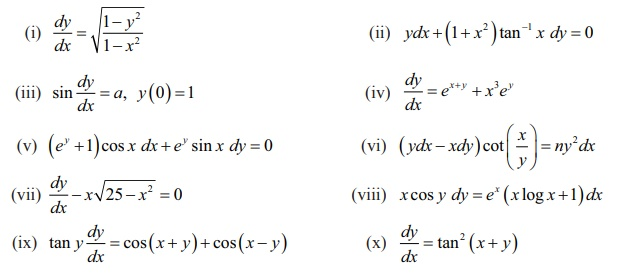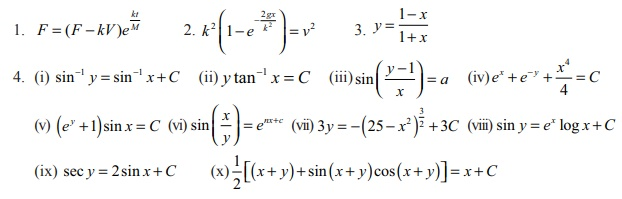Home | | Maths 12th Std | Exercise 10.5: Variables Separable Method, Substitution Method

# Exercise 10.5: Variables Separable Method, Substitution Method

Maths Book back answers and solution for Exercise questions - Mathematics : Ordinary Differential Equations: Solution of First Order and First Degree Differential Equations: Variables Separable Method, Substitution Method - Exercise Problem Questions with Answer, Solution

EXERCISE 10.5

1. If F is the constant force generated by the motor of an automobile of mass M , its velocity V is given by M dV/dt = F − kV , where k is a constant. Express V in terms of t given that = 0 when t = 0.2. The velocity v, of a parachute falling vertically satisfies the equationwhere g and k are constants. If v and x are both initially zero, find v in terms of x .3. Find the equation of the curve whose slope is y−1 / x2+x and which passes through the point (1, 0) .4. Solve the following differential equations: